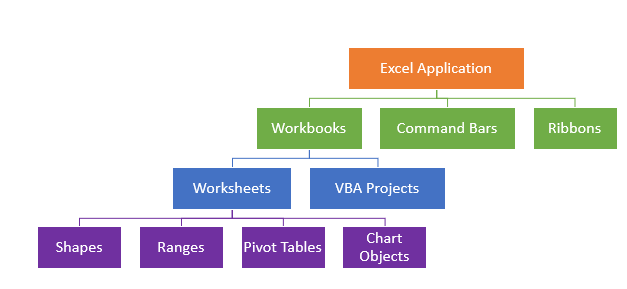# VBA Object Model

This tutorial will explain the VBA Object Model

Excel is made up of Objects – the Workbook Object, the Worksheet Object and the Range Object to mention just a few.  Each of these object are made up of Events, Properties and Methods that are able to be controlled by way of VBA code.

## The Excel Object Hierarchy

You can think of the Object Hierarchy in Excel, and in fact in any program within the Microsoft Office, as a bit like a family tree with the Application Object (Excel itself) being the sole parent object at the top of the tree.The top level – the Excel Application is a single object.  However, each of the subsequent levels can contain multiple objects.  Each of these levels can contain a COLLECTION of Objects – so Excel itself can contain many workbooks – a collection of workbooks.  Each workbook can then in turn contain many Worksheets, and each worksheet can then contain many different Ranges, Shapes, Pivot tables or Chart objects for example.  There are many more Objects available, but the graphic above will give you a general idea of how they all work together.

## Using Objects

Object are used in Sub-Procedures and Function Procedures to control the way Excel behaves.

The following procedure refers to a current workbook by using the Workbook Object.  It then creates a new worksheet in that workbook using the Worksheet Object.  Finally,  it uses the Range Object to bold and color a Range of cells in the worksheet.

``````Sub UsingObjects()
Dim wkb As Workbook
Dim wks As Worksheet
Dim rngA As Range
Dim rngB As Range
'set the objects
Set wkb = ActiveWorkbook
Set rngA = wks.Range("A1:E1")
Set rngB = wks.Range("A2:E20")
'maniuplate the range object by bolding and changing color
rngA.Font.Bold = True
rngB.Font.Color = -16776961
End Sub``````

Stop searching for VBA code online. Learn more about AutoMacro - A VBA Code Builder that allows beginners to code procedures from scratch with minimal coding knowledge and with many time-saving features for all users!